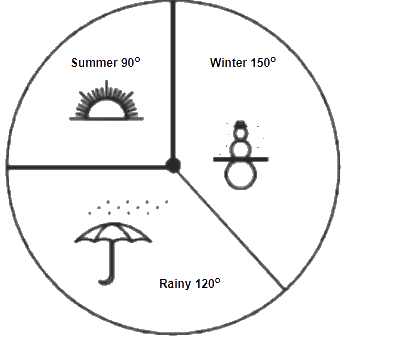# Ex.5.2 Q2 Data Handling Solutions - NCERT Maths Class 8

Go back to  'Ex.5.2'

## Question

A group of $$360$$ people were asked to vote for their favourite season from the three seasons rainy, winter and summer.

(i) Which season got the most votes?

(ii) Find the central angle of each sector.

(iii)Draw a pie chart to show this information.

 Season No. of votes Summer$$90$$ Rainy$$120$$ Winter$$150$$

Video Solution
Data Handling
Ex 5.2 | Question 2

## Text Solution

What is known?

Voting distribution of $$360$$ people for their favorite season.

What is unknown?

(i) The season got the most votes

(ii) The central angle of each sector

(iii) Pie chart that depicts this information

Reasoning:

A circle graph or pie chart shows the relationship between a whole and its parts.

Steps:

(i) By observing the given data,winter season got the most votes.

(ii) Central angle of summer

\begin{align} =\frac{90^{\circ} \times 360^{\circ}}{360^{\circ}}=90^{\circ} \end{align}

Central angle of rainy season

\begin{align} =\frac{120^{\circ} \times 360^{\circ}}{360^{\circ}}=120^{\circ} \end{align}

Central angle of winter season

\begin{align} =\frac{150^{\circ} \times 360^{\circ}}{360^{\circ}}=150^{\circ} \end{align}

(iii)Learn from the best math teachers and top your exams

• Live one on one classroom and doubt clearing
• Practice worksheets in and after class for conceptual clarity
• Personalized curriculum to keep up with school